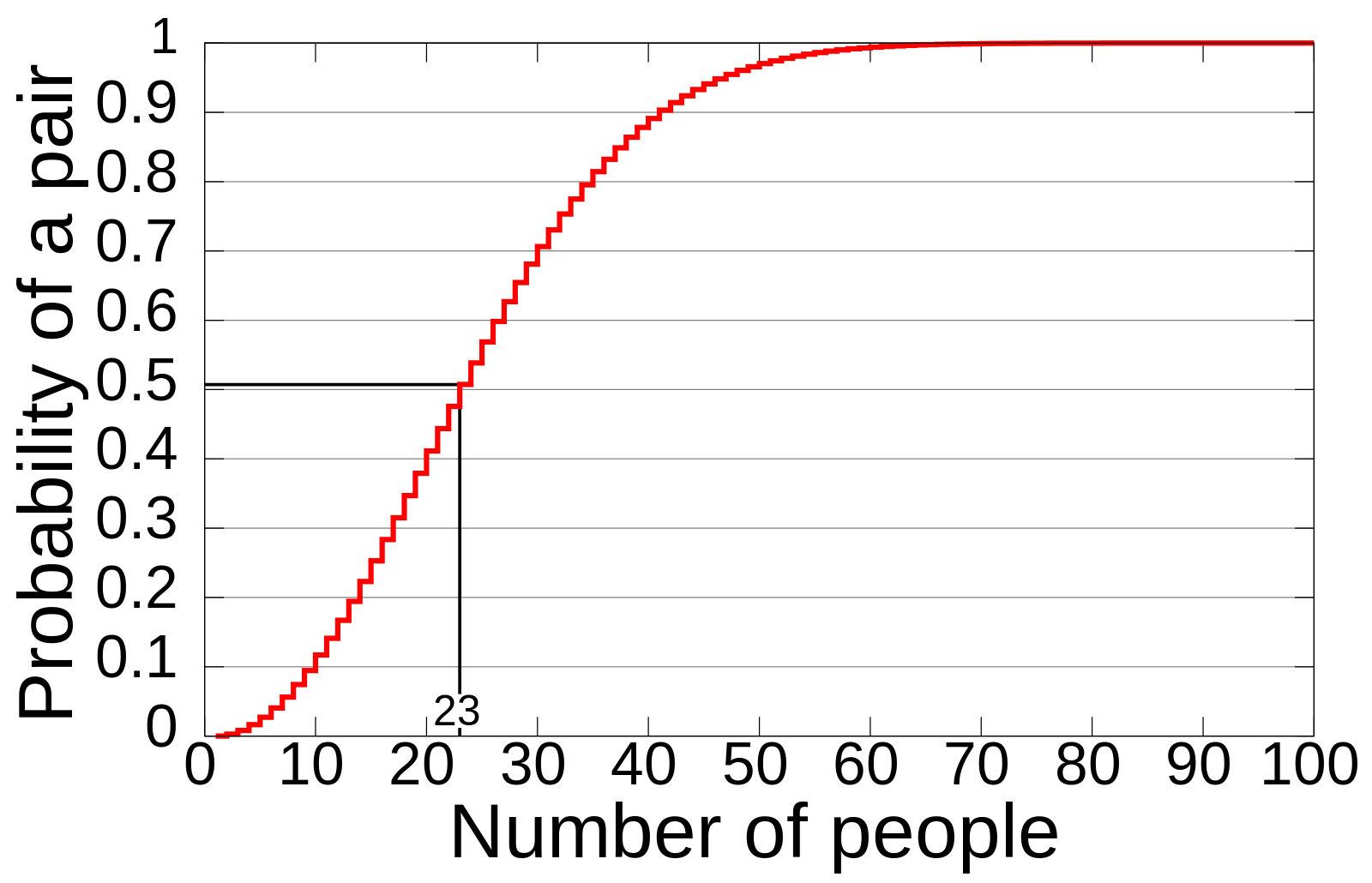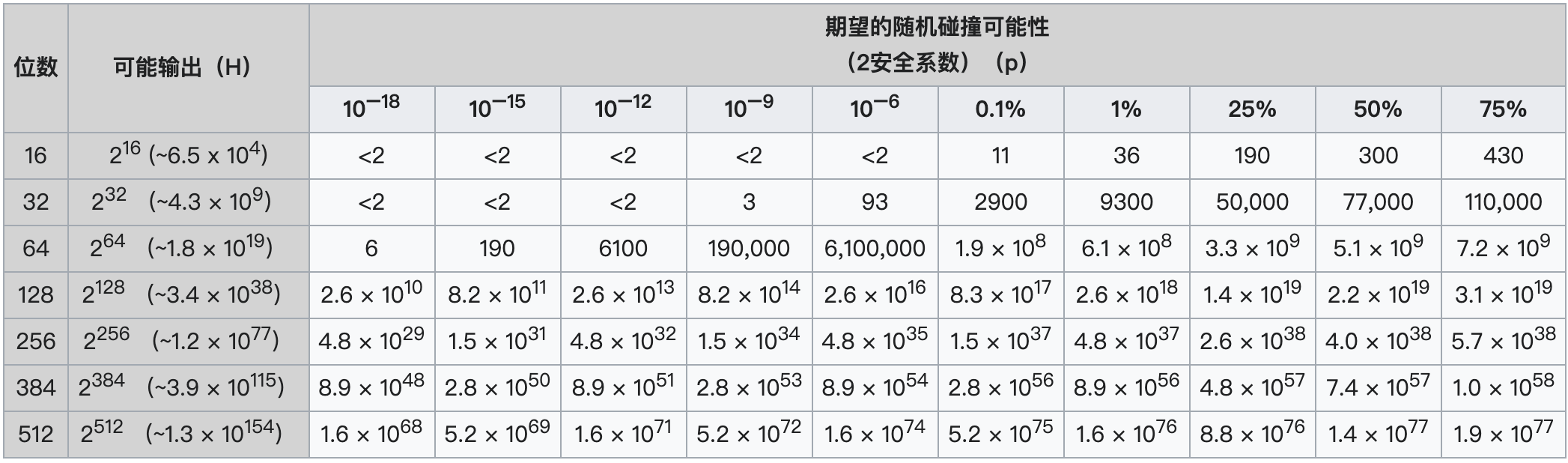## 哈希函数

• 用于哈希表的哈希函数。比如布隆过滤里的哈希函数，HashMap 的哈希函数。
• 用于加密和签名的哈希函数。比如，MD5，SHA-256。• 长度固定。任意的输入一定得到相同的输出长度。
• 确定性。相同的输入一定得到相同的输出。
• 单向性。通过输入得到输出，但是不能通过输出反推输入。

## 哈希函数质量

• 哈希值是否分布均匀，呈现出随机性，有利于哈希表空间利用率提升，增加哈希的破解难度；
• 哈希碰撞的概率很低，碰撞概率应该控制在一定范围；
• 是否计算得更快，一个哈希函数计算时间越短效率越高。

## 碰撞概率// 估算理论碰撞概率
public static double collisionProb(double n, double d) {
return 1 - Math.exp(-0.5 * (n * (n - 1)) / d);
}

//  估算达到碰撞概率时需要的哈希数量
public static long collisionN(double p, double d) {
return Math.round(Math.sqrt(2 * d * Math.log(1 / (1 - p))) + 0.5);
}

// 估算碰撞哈希数量
public static double collisionRN(double n, double d) {
return n - d + d * Math.pow((d - 1) / d, n);
}


1000万UUID，理论上的碰撞数量为11632.50

collisionRN(10000000, Math.pow(2, 32)) // 11632.50


private static Map<Integer, Set<String>> collisions(Set<String> values) {
Map<Integer, Set<String>> result = new HashMap<>();
for (String value : values) {
Integer hashCode = value.hashCode();
Set<String> bucket = result.computeIfAbsent(hashCode, k -> new TreeSet<>());
}
return result;
}

public static void main(String[] args) throws IOException {
Set<String> uuids = new HashSet<>();
for (int i = 0; i< 10000000; i++){
}
Map<Integer, Set<String>> values = collisions(uuids);

int maxhc = 0, maxsize = 0;
for (Map.Entry<Integer, Set<String>> e : values.entrySet()) {
Integer hashCode = e.getKey();
Set<String> bucket = e.getValue();
if (bucket.size() > maxsize) {
maxhc = hashCode;
maxsize = bucket.size();
}
}

System.out.println("UUID总数: " + uuids.size());
System.out.println("哈希值总数: " + values.size());
System.out.println("碰撞总数: " + (uuids.size() - values.size()));
System.out.println("碰撞概率: " + String.format("%.8f", 1.0 * (uuids.size() - values.size()) / uuids.size()));
if (maxsize != 0) {
System.out.println("最大的碰撞的字符串: " + maxsize + " " + values.get(maxhc));
}
}


UUID总数: 10000000



https://softwareengineering.stackexchange.com/questions/49550/which-hashing-algorithm-is-best-for-uniqueness-and-speed

## 小结

https://en.wikipedia.org/wiki/Birthday_problem